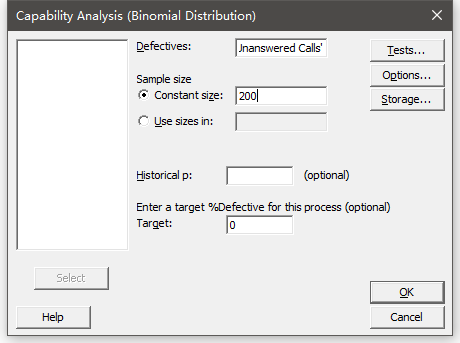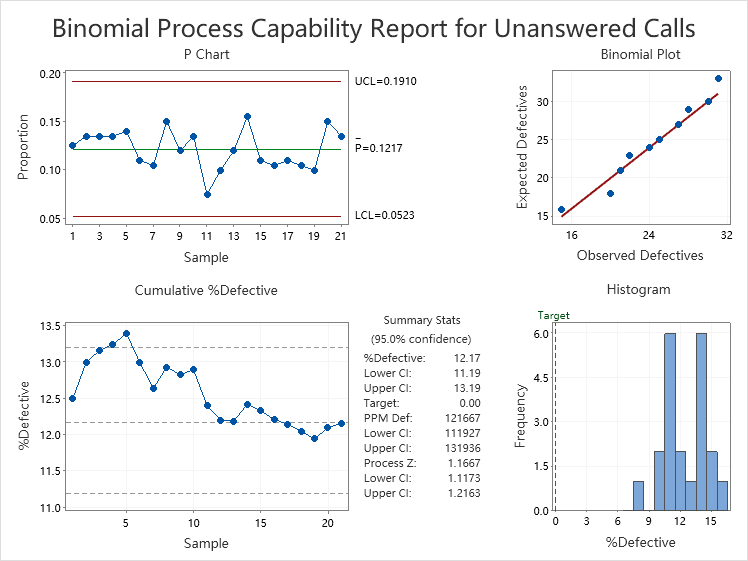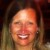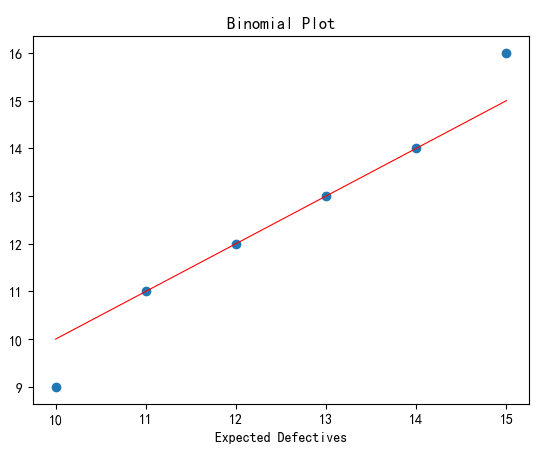# How to Get Expected Defectives in Minitab Binomial Capability Analysis

Six Sigma – iSixSigma Forums General Forums Tools & Templates How to Get Expected Defectives in Minitab Binomial Capability Analysis

Viewing 7 posts - 1 through 7 (of 7 total)
• Author
Posts
• #249351Anonymous
Inactive

I use minitab’s binomial capability analysis, and use the constant 200 in the Sample size.Minitab Result:Binomial Plot can be used to determine whether the data conforms to the binomial distribution.

It can be seen that the number of observed defects on the X axis is the category of the number of Unanswered Calls.
However, I don’t know how to calculate the expected number of defects on the Y axis.
I would like to know the specific calculation steps for the expected number of defects, thank you very much.

The data used is in the attachment.

0
#249357Katie Barry
Participant

@leavor Minitab has excellent technical support. Have you checked with them directly? I have notified them of this post in the meantime.

0
#249358MinitabUser1829
Participant

I have attached a document which gives the method for computing Expected Defectives.  An example is provided.  The screenshots are from an older version of Minitab, but the method is the same as what is in Minitab 19.

###### Attachments:
1. Instructions.docx
0
#249363Anonymous
Inactive

I have drawn Binomial Plot according to the provided help document

I am using Python and my code is as follows:

# -*- coding: utf-8 -*-
“””
Created on Thu Aug 13 11:20:54 2020
@author: leavor
“””
import pandas as pd
from collections import Counter
from scipy.stats import binom
from matplotlib import pyplot as plt
data = pd.Series([12,10,15,11,10,14,15,13,11,14,11,13,13,12,11,11,15,10,11,15,12,13,
12,13,14,12,13,13,10,12],name=’Defectives’)#Defectives
sampleSize = 20#sample size const
N = len(data)#N
collec = Counter(data)
key = list(collec.keys())#Observed Defectives
freq = list(collec.values())#Frequency
df = pd.DataFrame({‘OD’:key,’Freq’:freq})
df = df.sort_values(by=’OD’)
tmp = pd.Series().append(df[‘Freq’])
df[‘Indicator’] = tmp.cumsum().iloc[:-1].values#Indicator
df[‘Rank’] = (2*df[‘Indicator’]+df[‘Freq’]-1)/2#Rank
df[‘ECDF’] = (df[‘Rank’]-0.375)/(N+0.25)#ECDF
p = data.sum()/(N*sampleSize)#AverageProportion
df[‘ED’] = binom.ppf(df[‘ECDF’],sampleSize,p)#expected defectives
plt.scatter(df[‘OD’],df[‘ED’])
plt.plot(df[‘OD’],df[‘OD’],color=’r’,linewidth=0.8)
plt.xlabel(‘Observed Defectives’)
plt.xlabel(‘Expected Defectives’)
plt.title(‘Binomial Plot’)0
#249365Anonymous
Inactive

I tried to extend this method to the Poisson distribution, but encountered difficulties.

I don’t know the ECDF of Poisson calculation formula, and I don’t see the formula in the minitab supporting document.

@MinitabUser1829
,Can you provide a Poisson Plot method, thank you very much!

0
#249376MinitabUser1829
Participant

You can use the same steps as outlined in the document I attached before, with 2 changes:

• For step 5, calculate the average number of defects.
• For step 6, use Calc > Probability Distributions > Poisson.  For the Mean, enter the number computed from step 5.

0
#249390leavor
Participant

I’ve got it, thank you very much!

0
Viewing 7 posts - 1 through 7 (of 7 total)

You must be logged in to reply to this topic.